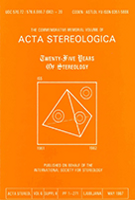Acta Stereologica -  Volume 11 (1992)  Number 2 - Dec. 1992

## Metrics, scalar product and correlation adapted to logarithmic images

Jean-Charles Pinoli
Pechiney, Centre de Recherches de Voreppe, B.P. 27, 38340 Voreppe, France

#### Abstract

Logarithmic images, such as images obtained by transmitted light or those outcoming from the human visual system, differ physically from linear images. So, their processing and analysis require specific mathematical laws and structures. The latter have been developed in the setting of the Logarithmic Image Processing model (Jourlin and Pinoli, 1985, 1987 and 1988). This model, called LIP, has already permitted the definition of theoretical notions such as the differentiation and the integration of logarithmic images (Pinoli, 1986 and 1987), and the well-justified introduction of perfoming practical notions : blending of two logarithmic images (Jourlin and Pinoli, 1988), contrast at a point or associated with a region or a boundary (Jourlin, Pinoli, Zeboudj, 1989 and Pinoli, 1991). Moreover, the LIP model appears to be an accurate framework for the introduction of other powerful and useful notions. The purpose of the present paper is to establish some of them : metrics, which appear to be naturally linked with the optical density concept, allowing the calculation of the distance between two logarithmic images, and also a scalar product allowing the introduction of the orthogonality and correlation concepts.

Keywords : correlation, image analysis, logarithmic images, metrics, orthogonality, scalar product